# Odoo 16 Development Book

## Float fields

The Float fields in Odoo are mainly used to store the numerical values or to store float values, and the null value is not supported. If there is no value for the field, then it returns 0.0. We might want to give the end user control over the decimal accuracy when using float fields. Here, we may add a Cost Price field with user-configurable decimal accuracy to the Library Books model. Maintaining the precise value of the float values is difficult (for example, 125.325). Additionally, the value of this kind of value varies depending on the platforms and environments.

### Decimal accuracy

A measurement tool called decimal accuracy enables the user to choose the floating location of various categories, such as a unit of measurement and pricing. Choosing how many values in each category should be shown as decimals is made much easier with the help of this feature. Let's use an example to go through this.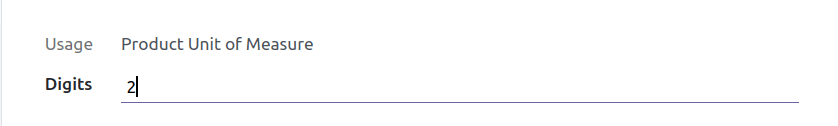Here, we can see that the decimal accuracy of the product unit of measure is 2, and looking at the product unit of measure.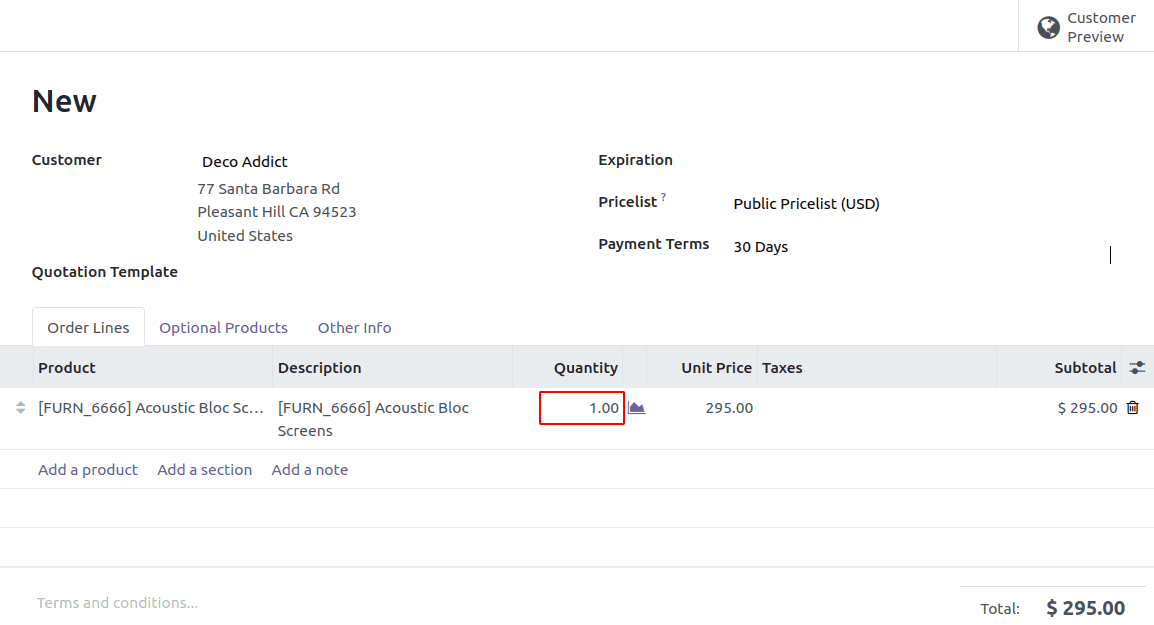Here we can see the decimal points are 2; When we give the decimal accuracy as five.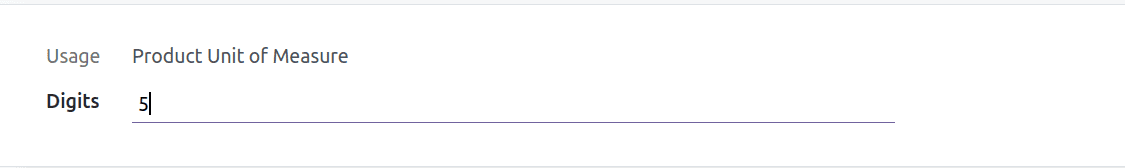Here we can see the decimal point as 5.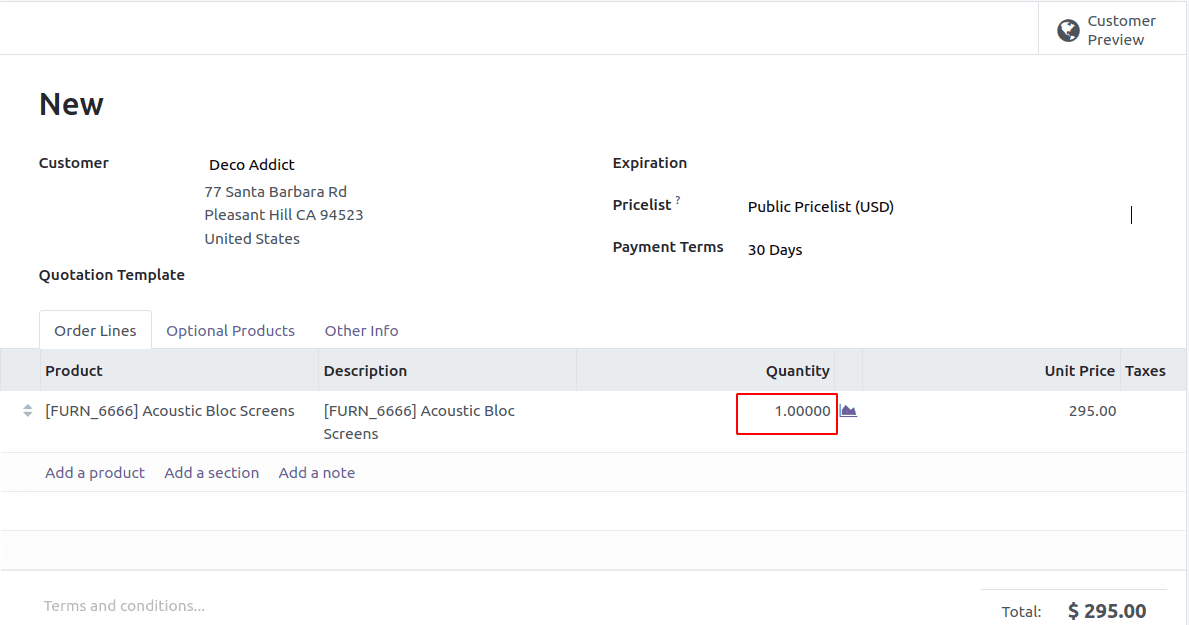We can create the float fields by code using the code below. At default, the decimal accuracy will be 2.

``fee = fields.Float(string='Fee')``

The result is,Odoo has some tools for determining the decimal location of a value. That is described further down.

1. float_compare(): The Odoo tools directory contains the float comparison function. This function is helpful when we need to compare two values.

2. float_is_zero(): This is a function that checks if a float is zero. If the given value can be considered zero using the precision value and rounding digits specified, this Odoo function returns true.

4. float_round(): This is also defined in float utils.py, which is located in the Odoo tools directory. After rounding the input value using the specified rounding parameters, this function returns the value that was supplied.

5. float_repr(): This method returns the textual representation of the given value with the chosen decimal precision. This is used to get the value of an afloat string. Rounding isn't a term used to describe it. It's possible that the erroneous value will be returned as a result of this. You can instead use the float round function ().

6. float_split_str(): This function is useful when you need to divide a float value from both an integer and a fraction value. It divides the given value accurately into its decimal and unitary parts. The float value is first rounded using the float round() function, which was previously covered. The rounded number is subsequently converted to a string using the float repr function (). A tuple will then be returned after the value has been divided into its integer and fractional components.

7. float_split(): This function is similar to float split str(), but instead of string values, it returns integer values.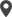Calicut

Cybrosys Technologies Pvt. Ltd.
Neospace, Kinfra Techno Park
Kakkancherry, Calicut
Kerala, India - 673635London

Cybrosys Limited
Alpha House,
100 Borough High Street, London,
SE1 1LB, United KingdomKochi

Cybrosys Technologies Pvt. Ltd.
1st Floor, Thapasya Building,
Kochi, India - 682030.Bangalore

Cybrosys Techno Solutions
The Estate, 8th Floor,